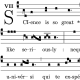## Composing array masks

Let’s say you have an array$P$, containing$N$ (~millions) points$P_i \in \mathbb{R}^3$. Perhaps it’s the output of an n-body simulation or something more complicated. Anyway, suppose you also have several other arrays of size$N$, each listing some quantity that is associated with each point$P_i$ – for example$M_i$, the mass at each point.

The task: find some small contiguous region$\Omega \subset \mathbb{R}^3$, and calculate some function at every point within it. For example, approximate the integral of the density by summing over the mass at each point:$\displaystyle \int_{\Omega} \rho(x) d^3x \approx \sum_{P_i \in \Omega} M_i$

Let us introduce an operation$A \star B = C$, where$|A| = |B|$,$B$ contains only zeroes or ones, and$C$ contains$A_i$ iff$B_i = 1$; also$|C| \leq |A|$, but$C$ maintains the order of elements from$A$. Assume that this operation can be calculated efficiently (in parallel), as opposed to sequentially traversing the elements of an array, which is much slower (this is the case in the IDL programming language, for example).

The solution to the problem at hand is to produce a mask$S$ containing$N$ elements with values the characteristic function$\chi_\Omega$ at each point – that is,$S_i = \chi_\Omega(P_i) = 1$ if$P_i \in \Omega$ and$0$ otherwise. Then the final calculation is$\displaystyle \sum_{P_i \in \Omega} M_i = \sum_i \left(M \star S\right)_i$

and the advantage is that we can reuse the mask$S$ for additional calculations.

Now suppose that$\Omega$ is itself a very large set, and that we have another, smaller region$\Omega' \subset \Omega$ on which we would also like to perform calculations. Let$P' = P \star S$ and$S' = \chi_{\Omega'}(P')$. Then we can compose our masks to find the$M$ values that lie within$\Omega'$.$\displaystyle \sum_{P_i \in \Omega'} M_i = \sum_i \left((M \star S) \star S'\right)_i.$

What are the$P$-indices of points that are in the region$\Omega'$? To find the answer we do:$\displaystyle A = [0,1,2,\ldots,|S|] \\ B = (A \star S) \star S'$

and$B$ now contains the desired$P$-indices.

In the IDL programming language,$A = B \star C$ is written as

A = B[where(C)]


A more general (and also efficient) way of dealing with large, unstructured lists of coordinates is to use an oct-tree.## About ejlflop

Intrepid explorer of music, mathematics, computer programming. physics (an unordered list). Enthusiastic semi-lay-person.
This entry was posted in maths, programming. Bookmark the permalink.